## Example Questions

### Example Question #1 : How To Subtract Odd Numbers

Tom has twice as many chores as Jeff, who has 3 less than Steve.  Steve has 10 chores. How many chores does Tom have?Explanation:

If Steve has 10 chores, Jeff has 7 chores (3 less than Steve).  Tom has twice as many as Steve, so Tom has 14 chores.

### Example Question #2 : How To Subtract Odd Numbers

Your mom made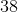cookies. You ate, your brother ate twice as many as you did, and your sister ate. How many are left?Explanation:

Create an equation for the situation described and solve: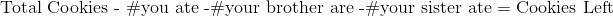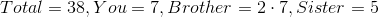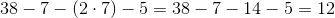### Example Question #3 : How To Subtract Odd Numbers

Your mom gives outtotal presents each year to your cousins. If your cousin Sally receivedand your cousin Billy receivedtimes as many as Sally, how many did your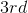cousin, Becky get?Explanation:

Create an equation to decribe the above situation and solve.

Total presents given out is represented by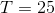.

Presents given to Sally is represented by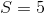.

Presents given to Billy is represented by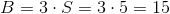.

Thus our equation becomes,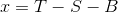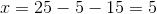### Example Question #4 : How To Subtract Odd Numbers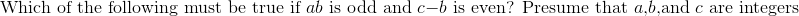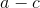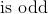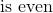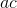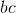Always start by gathering your facts. You know that if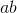is odd, then neither of those numbers are even. (If either were, then the product would be even.)  Now, the rules for subtracting are just like adding. If the difference of two numbers is even, then they must either both be odd or both be even. Thus if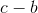is even and we know thatis odd, then we know thatmust be odd. Thus ifis odd, it is also true thatis even, for bothandare even.